### python3+dlib实现人脸识别和情绪分析

1、嘴巴张开距离占面部识别框宽度的比例越大，说明情绪越激动，可能是非常开心，也可能是极度愤怒。

2、眉毛上扬，17-21 或者 22-26 号特征点距离面部识别框顶部与识别框高度的比值越小，说明眉毛上扬越厉害，可表示惊讶、开心。眉毛的倾斜角度，开心时眉毛一般是上扬，愤怒时皱眉，同时眉毛下压的比较厉害。

3、眯眼睛，人在开怀大笑的时候会不自觉的眯起眼睛，愤怒或者惊讶的时候会瞪大眼睛。

1、安装VS2015，因为最新版的dlib-19.10需要这个版本的vscode

2、安装opencv（whl方式安装）：

3、安装dlib（whl方式安装）：

dlib各种版本的whl文件：https://pypi.python.org/simple/dlib/

4、如果想要使用人脸模型特征标定的话，还需要一个人脸面部形状预测器，这个可以通过自己的照片进行训练，也可以使用dlib作者给出的一个训练好的预测器：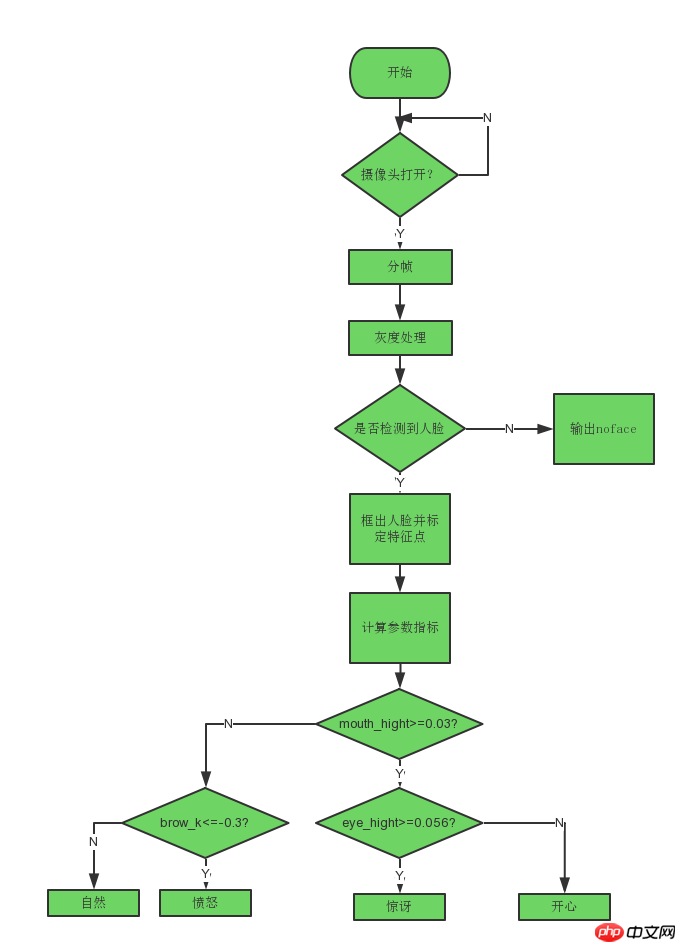```import cv2
import dlib
from skimage import io

# 使用特征提取器get_frontal_face_detector
detector = dlib.get_frontal_face_detector()
# dlib的68点模型，使用作者训练好的特征预测器
predictor = dlib.shape_predictor("shape_predictor_68_face_landmarks.dat")
# 图片所在路径
# 生成dlib的图像窗口
win = dlib.image_window()
win.clear_overlay()
win.set_image(img)

# 特征提取器的实例化
dets = detector(img, 1)
print("人脸数：", len(dets))

for k, d in enumerate(dets):
print("第", k+1, "个人脸d的坐标：",
"left:", d.left(),
"right:", d.right(),
"top:", d.top(),
"bottom:", d.bottom())

width = d.right() - d.left()
heigth = d.bottom() - d.top()

print('人脸面积为：',(width*heigth))```

``` # 利用预测器预测
shape = predictor(img, d)
# 标出68个点的位置
for i in range(68):
cv2.circle(img, (shape.part(i).x, shape.part(i).y), 4, (0, 255, 0), -1, 8)
cv2.putText(img, str(i), (shape.part(i).x, shape.part(i).y), cv2.FONT_HERSHEY_SIMPLEX, 0.5, (255, 255, 255))
# 显示一下处理的图片，然后销毁窗口
cv2.imshow('face', img)
cv2.waitKey(0)```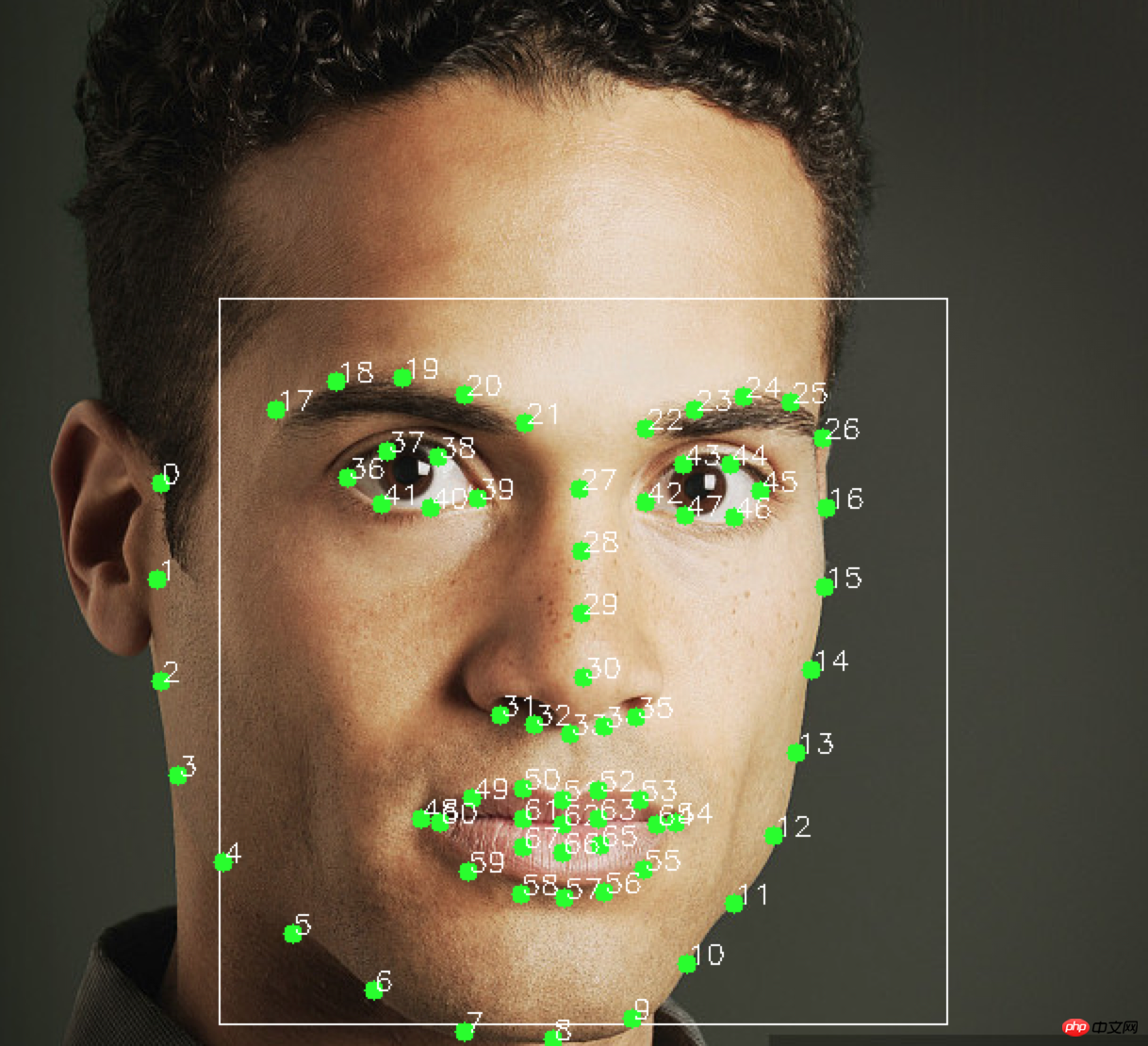```# 眉毛
brow_sum = 0  # 高度之和
frown_sum = 0  # 两边眉毛距离之和
for j in range(17,21):
brow_sum+= (shape.part(j).y - d.top()) + (shape.part(j+5).y- d.top())
frown_sum+= shape.part(j+5).x - shape.part(j).x
line_brow_x.append(shape.part(j).x)
line_brow_y.append(shape.part(j).y)

self.excel_brow_hight.append(round((brow_sum/10)/self.face_width,3))
self.excel_brow_width.append(round((frown_sum/5)/self.face_width,3))
brow_hight+= (brow_sum/10)/self.face_width    # 眉毛高度占比
brow_width+= (frown_sum/5)/self.face_width    # 眉毛距离占比

tempx = np.array(line_brow_x)
tempy = np.array(line_brow_y)
z1 = np.polyfit(tempx, tempy, 1) # 拟合成一次直线
self.brow_k = -round(z1, 3)  # 拟合出曲线的斜率和实际眉毛的倾斜方向是相反的```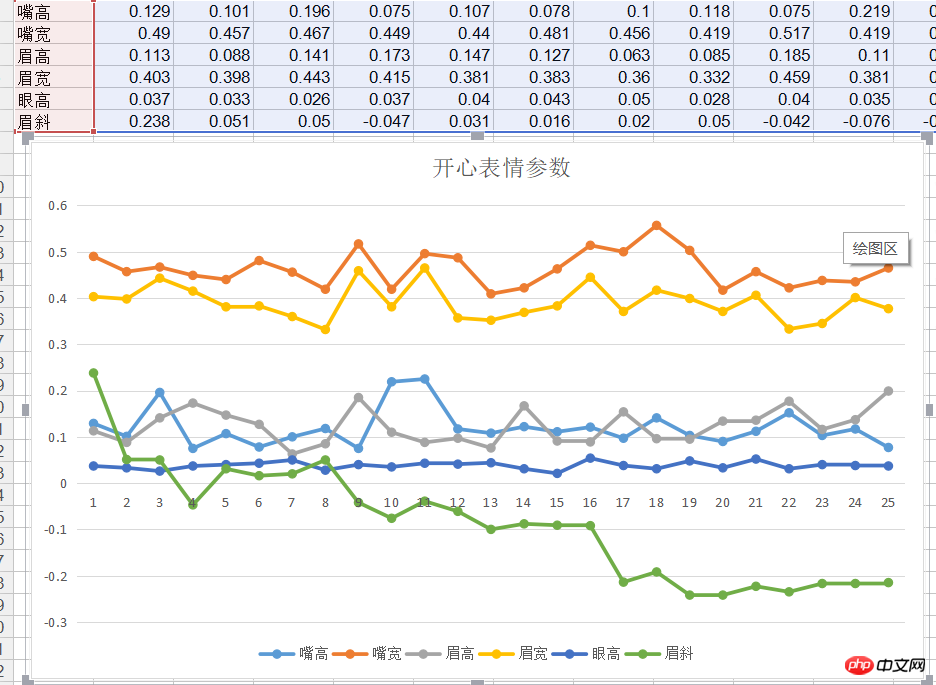```# 分情况讨论
# 张嘴，可能是开心或者惊讶
if round(mouth_higth >= 0.03):
if eye_hight >= 0.056:
cv2.putText(im_rd, "amazing", (d.left(), d.bottom() + 20), cv2.FONT_HERSHEY_SIMPLEX, 0.8,
(0, 0, 255), 2, 4)
else:
cv2.putText(im_rd, "happy", (d.left(), d.bottom() + 20), cv2.FONT_HERSHEY_SIMPLEX, 0.8,
(0, 0, 255), 2, 4)

# 没有张嘴，可能是正常和生气
else:
if self.brow_k <= -0.3:
cv2.putText(im_rd, "angry", (d.left(), d.bottom() + 20), cv2.FONT_HERSHEY_SIMPLEX, 0.8,
(0, 0, 255), 2, 4)
else:
cv2.putText(im_rd, "nature", (d.left(), d.bottom() + 20), cv2.FONT_HERSHEY_SIMPLEX, 0.8,
(0, 0, 255), 2, 4)```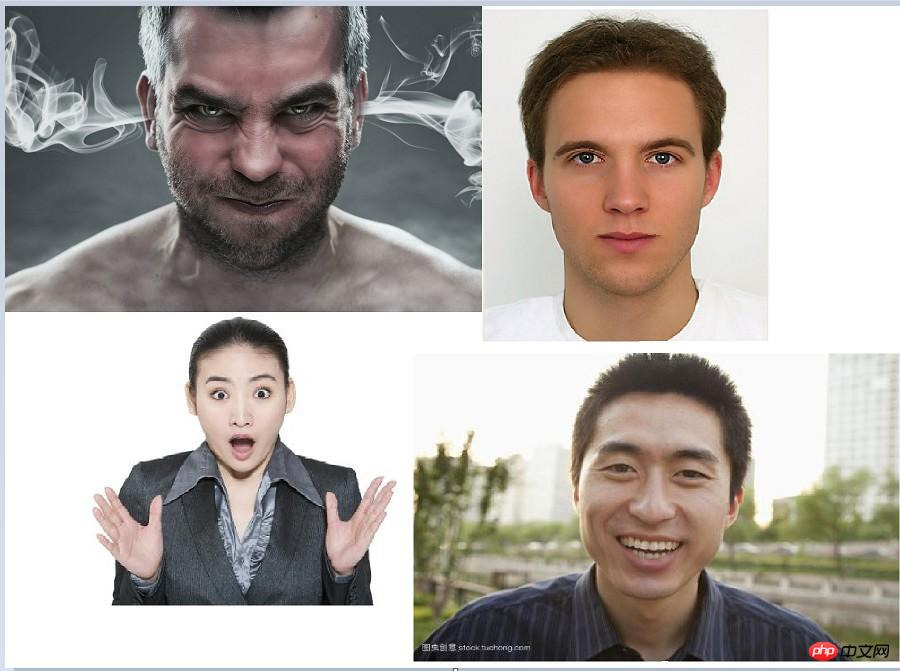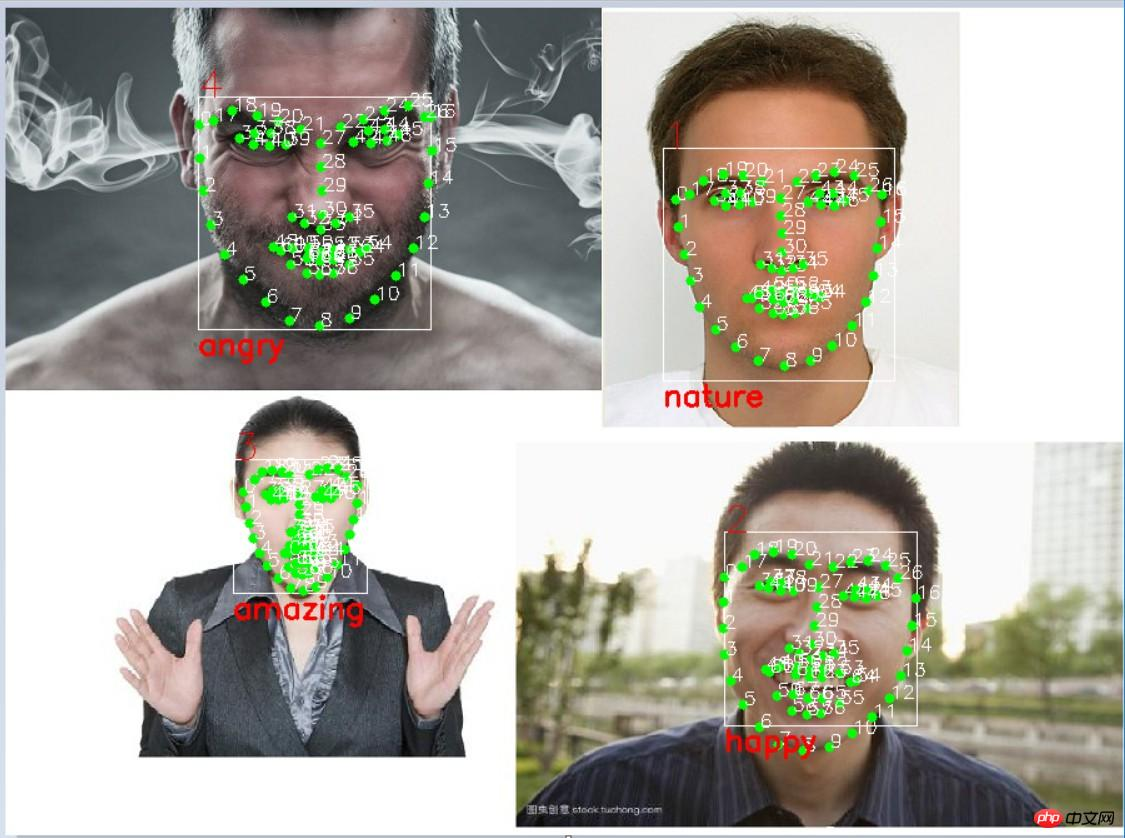### 每日一笑关闭### 给这篇文章打个标签吧~

棒极了 糟糕透顶 好文章 PHP JAVA JS 小程序 Python SEO MySql 确认